• 一维线性插值算法
2021-03-15 15:59:25

插值算法有多项式插值、艾尔米特插值、分段插值与样条插值、三角函数插值、辛克插值等等

在MATLAB中用函数interp1()函数来进行一维值,代码如下：

clear  clc;

x=0:2*pi;

y=sin(x);

xx=0:0.5:2*pi;

yy=interp1(x,y,xx);

plot(x,y,'s',xx,yy);

interp1(X,Y,Xq,METHOD):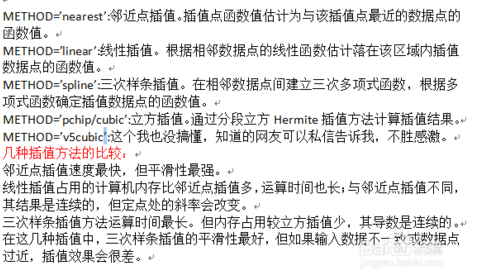一维快速傅立叶插值，matlab使用intepft(x,n)函数来实现一维快速傅立叶插值。该函数用傅立叶变换把输入数据变换到频域，然后用更多点的傅立叶逆变换变回时域，来实现对数据的增采样。

y=intepft(x,n，dim):对x进行傅立叶变换，然后采用n点傅立叶逆变换变回到时域，如果x是一个向量，数据x的长度为m,采样间隔为dx,则数据y的采样间隔是mdx/n(m

下面我们通过一个简单的一个简单的例子来进一步说明：

%使用一维快速傅立叶插值实现指定函数的数据增采样

clear clc;

x=1:10;

y=exp(x);

%实现一倍增采样

n=2*length(x);

yi=interpft(y,n);

xi=1:0.5:10.5;

hold on;

plot(x,y,'ro');

plot(xi,yi,'b*-');

title('一维快速傅立叶插值');

legend('原始数据','插值结果');

在某些特定情况下，一维快速傅立叶插值法有奇效哦。最后提一点，当数据点呈现周期分布时，上面几种插值算法的误差很大，此时可采用快速fourier算法.

更多相关内容
• 1、面向一维插值和线性拟合，含有牛顿插值、线性插值等四种插值方式； 2、设计了可用鼠标直接操作的GUI界面
• 3D图像线性插值算法实现 三线性插值原理 三线性插值原理与双线性插值原理类似，双线性插值是根据周围四个点计算得到新的插值点，三线性插值是对个立方体中周围8个顶点进行计算。 1）首先在x轴上进行插值，根据...

## 3D图像线性插值算法实现

1. 三线性插值原理
三线性插值原理与双线性插值原理类似，双线性插值是根据周围四个点计算得到新的插值点，三线性插值是对一个立方体中周围8个顶点进行计算。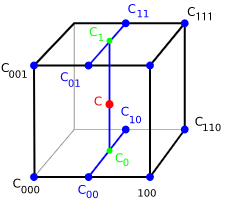1）首先在x轴上进行插值，根据以下公式分别求出4个边上的点C00，C01，C10，C11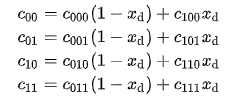2）在y轴上进行插值，根据一下公式得到C0和C1点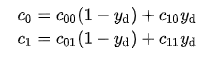3）最后在z轴上进行插值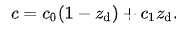其中xd， yd，zd是指偏移量，与x，y，z较小的坐标有关。xd = x - |xd|
实际上三线性插值等同于两个双线性插值与线性插值的组合。当前这样计算起来很麻烦，所以使用另一个方案进行编写插值算法。

1. 替代算法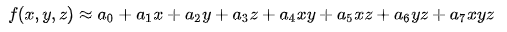求解方程线性找到系数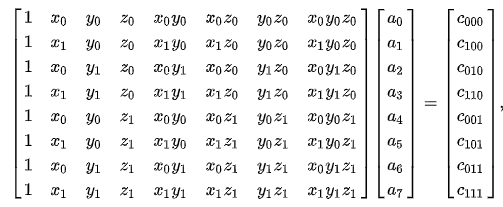直接看结果吧，反正我也推不出来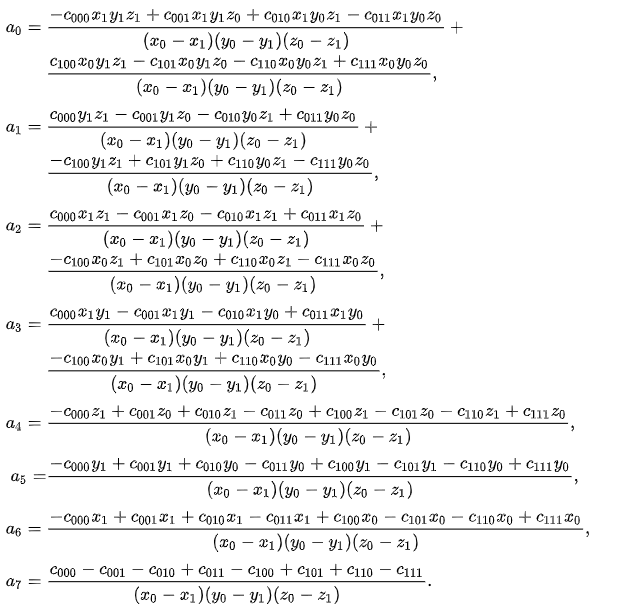2. 算法实现
import numpy as np
import math

def Interpolation3D(src_img, dst_size)：
#srcImage原3d图像，dst_size插值后图像的大小
srcZ, srcY, srcX = src_img.shape   #原图图像大小
dst_img = np.zeros(shape = dst_size, dtype = np.int16)  #插值后的图像

new_Z, new_Y, new_X = dst_img.shape  #
print("插值后图像的大小", dst_img.shape)

factor_z = srcZ / new_Z
factor_y = srcY / new_Y
factor_x = srcX / new_X

for z in range(new_Z):
for y in range(new_Y):
for x in range(new_X):

src_z = z * factor_z
src_y = y * factor_y
src_x = x * factor_x

src_z_int = math.floor(z * factor_z)
src_y_int = math.floor(y * factor_y)
src_x_int = math.floor(x * factor_x)

w = src_z - src_z_int
u = src_y - src_y_int
v = src_x - src_x_int

# 判断是否查出边界
if src_x_int + 1 == dimX or src_y_int + 1 == dimY or src_z_int + 1 == dimZ:
dst_img[z, y , x] = src_img[src_z_int, src_y_int, src_x_int]

else:

C000 = src_img[src_z_int, src_y_int, src_x_int]
C001 = src_img[src_z_int, src_y_int, src_x_int + 1]
C011 = src_img[src_z_int, src_y_int + 1, src_x_int + 1]
C010 = src_img[src_z_int, src_y_int + 1, src_x_int]
C100 = src_img[src_z_int + 1, src_y_int, src_x_int]
C101 = src_img[src_z_int + 1, src_y_int, src_x_int + 1]
C111 = src_img[src_z_int + 1, src_y_int + 1, src_x_int + 1]
C110 = src_img[src_z_int + 1, src_y_int + 1, src_x_int]
dst_img[z ,y ,x] = C000 * (1 - v)* (1 - u) * (1 - w) + \
C100 * v * (1 - u) * (1 - w) + \
C010 * (1- v) * u * (1 - w) + \
C001 * (1 - v) * (1 - u) * w + \
C101 * v * (1 - u) * w + \
C011 * (1 - v) * u * w + \
C110 * v * u * (1 - w) + \
C111 * v * u * w

return dst_img


展开全文python
• ## 二维线性插值方法

千次阅读 2021-12-21 22:57:08
​ 前几天在进行数据仿真的时候,对于将表格离散数据转化成连续数据一直是件十分棘手的事情，在网站上找了许多资源最后才找到可以利用二维线性插值的方法将数据进行转化。 1.原理 ​ 是要将m×nm\times nm×n的二维...

​ 前几天在进行数据仿真的时候,对于将表格离散数据转化成连续数据一直是一件十分棘手的事情，在网站上找了许多资源最后才找到可以利用二维线性插值的方法将数据进行转化。

### 1.原理

​ 是要将 m × n m\times n 的二维数据如下图所示进行拟合操作找出 C = f ( M a , α ) C=f(Ma,\alpha) 的插值式。在这里我们假设对表格中的一个 2 × 2 2 \times 2 的数据进行插值操作,其基本系数描述如下:
f ( M a , α ) = a 0 + a 1 M a + a 2 α + a 3 M a × α f(Ma,\alpha)=a_0+a_1Ma+a_2\alpha+a_3Ma\times\alpha
在已知四个坐标 ( x 1 , y 1 , f 1 ) , ( x 2 , y 2 , f 2 ) , ( x 3 , y 3 , f 3 ) , ( x 4 , y 4 , f 4 ) (x_1,y_1,f_1),(x_2,y_2,f_2),(x_3,y_3,f_3),(x_4,y_4,f_4) 为插值点的情况下可以得到这四个坐标点区域内的拟合曲面的系数 ( α 0 , α 1 , α 2 , α 3 ) T (\alpha_0,\alpha_1,\alpha_2,\alpha_3)^T :
( 1 x 1 y 1 x 1 y 1 1 x 2 y 2 x 2 y 2 1 x 3 y 3 x 3 y 3 1 x 4 y 4 x 4 y 4 ) ( a 0 a 1 a 2 a 3 ) = ( f 1 f 2 f 3 f 4 ) A x = b → x = A − 1 b \begin{pmatrix} 1 & x_1&y_1&x_1y_1\\1 & x_2&y_2&x_2y_2\\1 & x_3&y_3&x_3y_3\\1 & x_4&y_4&x_4y_4\\\end{pmatrix}\begin{pmatrix} a_0\\a_1\\a_2\\a_3\\\end{pmatrix}=\begin{pmatrix} f_1\\f_2\\f_3\\f_4\\\end{pmatrix}\\ Ax=b\rightarrow x = A^{-1}b

### 2.代码

例如对下面的表格中的数据进行插值:主函数:

clc,clear;close all;
X = 0.9;Y = 9*pi/180;
CX = [0.4171 0.3858 0.3779 0.3785 0.3787 0.3829 0.3855 0.4082 0.4947;...
0.4404 0.4086 0.4007 0.4015 0.4018 0.4062 0.4091 0.4321 0.5192;...
0.5219 0.4903 0.4827 0.4838 0.4846 0.4897 0.4934 0.5175 0.6073;...
0.6603 0.6290 0.6218 0.6234 0.6249 0.6310 0.6363 0.6621 0.7571;...
0.8534 0.8826 0.8160 0.8184 0.8209 0.8284 0.8358 0.8641 0.9672;...
1.1023 1.0723 1.0666 1.0700 1.0738 1.0835 1.0938 1.1254 1.2392];
%X,Y是想求的点处的位置
y = linear_cha(CX,X,Y);

%下面对表格中的数据进行线性插值函数
function y = linear_cha(CX,X,Y)
ma = 0.1:0.1:0.9;alpha = (0:2:10)*pi/180;
%寻找横坐标
for i = 1:8
if (ma(i)<X)&&(ma(i+1)>=X)
X_ = ma(i);
index_i = i;
break;
end
end
%寻找纵坐标
for i = 1:5
if (alpha(i)<Y)&&(alpha(i+1)>=Y)
Y_ = alpha(i);
index_j = i;
break;
end
end
x1 = ma(index_i);y1 = alpha(index_j);
x2 = ma(index_i);y2 = alpha(index_j + 1);
x3 = ma(index_i + 1);y3 = alpha(index_j + 1);
x4 = ma(index_i + 1);y4 = alpha(index_j);
%构造多项式矩阵求解系数
A = [1 x1 y1 x1*y1;...
1 x2 y2 x2*y2;...
1 x3 y3 x3*y3;...
1 x4 y4 x4*y4];
b = [CX(index_j,index_i);...
CX(index_j+1,index_i);...
CX(index_j+1,index_i+1);...
CX(index_j,index_i+1)];
x = A\b;
y = x'*[1;X;Y;X*Y];
end

展开全文机器学习 人工智能
• MATLAB 提供了 interp1(x,y,xq,'Method')函数命令可以进行一维插值，其中一维插值有四种常用的方法，也就是 ‘Method’ 可以选择邻近点插值Nearest，线性插值Linear，三次样条插值Spline和立方插值Pchip。...

MATLAB 提供了 interp1(x,y,xq,'Method')函数命令可以进行一维插值，其中一维插值有四种常用的方法，也就是 ‘Method’ 可以选择邻近点插值Nearest，线性插值Linear，三次样条插值Spline和立方插值Pchip

## 插值效果

#### 邻近点插值（Nearest）#### 线性插值（Linear）线性插值法是指使用连接两个已知量的直线来确定在这两个已知量之间的一个未知量的值的方法。

#### 三次样条插值（Spline）三次样条插值（Cubic Spline Interpolation）简称Spline插值，是通过一系列形值点的一条光滑曲线，数学上通过求解三弯矩方程组得出曲线函数组的过程。

实际计算时还需要引入边界条件才能完成计算。一般的计算方法书上都没有说明非扭结边界的定义，但数值计算软件如Matlab都把非扭结边界条件作为默认的边界条件。

早期工程师制图时，把富有弹性的细长木条（所谓样条）用压铁固定在样点上，在其他地方让它自由弯曲，然后沿木条画下曲线。成为样条曲线。

#### 立方插值（Pchip）## 实验代码

下面对MATLAB这四种一维插值（interp1）的方法进行比较。

close all; clear all; clc

x=0:0.5:2*pi;   % 插值前的矩阵规模
y=sin(x);

%-1-邻近点插值--Nearest interpolation---%
figure('Position',[50,50,500,400],'Name','Nearest');
x1=0:0.1:2*pi;    % 插值后的矩阵规模
y1=interp1(x,y,x1,'Nearest');
plot(x,y,'.','color','r','MarkerSize',20);  hold on;
plot(x1,y1,'.','color','b','MarkerSize',10);    hold off;
xlabel('x','fontsize',20);
ylabel('y','fontsize',20);

%-2-线性插值--Linear interpolation---%
figure('Position',[100,100,500,400],'Name','Linear');
x2=0:0.1:2*pi;
y2=interp1(x,y,x1,'Linear');
plot(x,y,'.','color','r','MarkerSize',20);  hold on;
plot(x2,y2,'.','color','b','MarkerSize',10);    hold off;
xlabel('x','fontsize',20);
ylabel('y','fontsize',20);

%-3-三次样条插值--Spline interpolation---%
figure('Position',[150,150,500,400],'Name','Spline');
x3=0:0.1:2*pi;
y3=interp1(x,y,x1,'Spline');
plot(x,y,'.','color','r','MarkerSize',20);  hold on;
plot(x3,y3,'.','color','b','MarkerSize',10);    hold off;
xlabel('x','fontsize',20);
ylabel('y','fontsize',20);

%-4-立方插值--Pchip interpolation---%
figure('Position',[200,200,500,400],'Name','Pchip');
x4=0:0.1:2*pi;
y4=interp1(x,y,x1,'Pchip');
plot(x,y,'.','color','r','MarkerSize',20);  hold on;
plot(x4,y4,'.','color','b','MarkerSize',10);    hold off;
xlabel('x','fontsize',20);
ylabel('y','fontsize',20);

展开全文matlab
• C++实现复数的一维线性插值interp1导言函数需求分析源码注意 导言 最近在进行Qt开发，涉及大量的matlab转C的工作，其中包括插值滤波等，这里对matlab的interp1函数进行了研究并用C++进行了重写。 经对比，结果与...matlab
• program main implicit none ...// x与y的数据来源于matlab的interp1帮助文档, t为被插值节点，见帮助文档的变量xq x = [0.d0,0.785398163397448d0,1.57079632679490d0,2.35619449019235d0,3.14159265358979d0.
• 三线性插值（trilinear interpolation）主要是用于在个3D的立方体中，通过给定顶点的数值然后计算立方体中其他点的数值的线性插值方法。 具体推导过程见参考资料1，这里直接给出最终公式： 其中，坐标(x,y,z...线性插值
• interpl( )一维插值函数 格式： yi＝interpl (x, y, xi, 'method') 功能：为给定的数据对(x,y)以及x坐标上的插值范围向量xi，用指定所使用的插值方法method实现插值。yi是插值后的对应数据点集的y坐标 简单来...matlab 插值函数 线性插值
• 通过分别介绍拉格朗日插值法、分段线性插值法和三次样条插值法的理论知识，结合数学软件Matlab编程工具，对机床加工零件问题展开研究。针对3种插值方法所得到的图像曲线，比较它们的适宜性，分析和判断哪条曲线最...
• 线性插值法：是指使用连接两个已知量的直线来确定在这两个已知量之间的个未知量的值的方法。 线性插值相比其他插值方式，如抛物线插值，具有简单、方便的特点。 线性插值可以用来近似代替原函数，也可以用来计算...线性插值
• 考虑这个y(x)函数：我们可以在文件中生成这些分散的点：dataset_1D.dat：# x y0 01 12 03 -94 -32以下是这些点的一维插值代码：加载这些分散的点创建x_mesh执行1D插值代码：^{pr2}\$图中显示了以下内容：现在，考虑这...
•fpga开发 计算机视觉
• 线性插值 先介绍线性插值的概念。 已知两个点(x1, y1)、(x2, y2)，求它们中间横坐标为x的点的y值。 则可以利用如下公式进行插值计算。其中a和(1-a)为x距离x1和x2的距离占(x2-x1)的比例。 y = a*y1 + (1-a)*y2...数字图像处理
• 拉格朗日插值法： 可以用于少样本点的情况，一定可以返回较好的插值函数。当样本点较多时，函数复杂度急剧上升。 样条插值法： 低阶多项式进行拟合，误差比拉格朗日稍大，但是避免了龙格现象。 ...matlab python
• %双三次插值具体实现clc,clear;fff=imread('E:\Documents\BUPT\DIP\图片\lena.bmp');ff =rgb2gray(fff);%转化为灰度图像[mm,nn]=size(ff);%将图像隔行隔列抽取元素，得到缩小的图像fm=mm/2;n=nn/2;f =zeros(m,n);for...
• ## C# 线性插值

千次阅读 2021-01-01 11:51:05
1. 对于给定起点、终点和插值点数目，生成线性插值后的数组 2.对于缺失部分值得数据序列，进行线性插值，补充该序列（如有组数据中间缺失了部分，用其起点和终点进行线性插值
• 函数功能：输入两个值，制定插值后数组长度，输出线性插值后的数组。 void test(double a, double b){ cout << "section: " << a << " " << b << endl; const int ...
•Matab
• 文章目录创建项目拉格朗日插值 创建项目 使用VS2019创建C++控制台应用 C++菜鸟教程 拉格朗日插值 https://blog.csdn.net/u013871100/article/details/41249285/ ...拉格朗日插值 牛顿插值 分段线性插值
• ## 插值算法

千次阅读 2021-03-13 09:00:21
老师讲得很详细，很受用！！！作用数模比赛中，常常需要根据已知的函数点...一维插值问题定义方法分类本文重点介绍数学建模常用的两种方法：三次样条插值和分段三次埃尔米特插值插值多项式原理拉格朗日插值法方法...
• 官方文档链接：https://docs.scipy.org/doc/scipy-1.3.0/reference/generated/scipy.interpolate.interp1d.html#scipy.interpolate.interp1dscipy库中可以通过interp1d类来实现一维插值照例还是官方文档的翻译与解释...
• 需要做7次一维线性插值 4次线性插值得到I、J、K、LI、J、K、LI、J、K、L 2次线性插值得到M、NM、NM、N 1次线性插值得到OOO 设(O−A)=(u,v,w)(O-A) = (u,v,w)(O−A)=(u,v,w)， u v w 就相当于权重 I=A+u(B−A)J=...
• 3.1 一维插值方法 1、数据处理的应用背景 2、插值的基本原理 3、引例 3.1、引例演示 4、一维插值定义 5、一维插值原理 6、一维插值方法 6.1、拉格朗日插值 6.2、分段线性插值 6.3、三次样条插值 3.2 二维插值方法 1...matlab 数据拟合
• 目录 1.关于插值： ...线性插值是针对一维数据的插值方法。它根据一维数据序列中需要插值的点的左右临近两个数据来进行数值估计。当然了它不是求这两个点数据大小的平均值（在中心点的时候就等于平均...python
• matlab代码二次插值自述文件（reduce-linear-interp1） 对于指定的绝对误差容限，请减少与MATLAB中的线性插值（interp1）一起使用的1D数据集。 ...但是，此提交仅限于一维线性插值，而其他提交则适
• 各位博友们大家好，小弟遇到一些问题经常会去看大家的博客，所以也想加入大伙的阵营，每每看到一些好的东西，有些心得体会什么的也... 基于这样种功能，我目前知道的应用是：1）它可以对数据中的缺失进行合理的补...
• 文章目录、认识插值二、拉格朗日插值三、分段线性插值四、三次样条插值 、认识插值 插值的分类： 拉格朗日插值（高次多项式插值）： 其插值函数在整个区间上是个解析表达式，便于再次开发利用； 曲线光滑......